Home > GC > Chapter 8 > Lesson 8.3.1 > Problem8-89

8-89.
1. On graph paper, plot the points A(−3, −1) and B(6, 11). 8-89 HW eTool (Desmos). Homework Help ✎

1. Find the midpoint of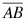.

2. Find the equation of the line that passes through points A and B.

3. Find the distance between points A and B.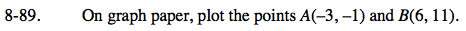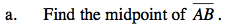$\text{Calculate the midpoint. }\left ( \frac{x_{1}+x_{2}}{2},\frac{y_{1}+y_{2}}{2} \right )$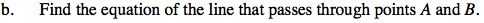What is the y-intercept? What is the slope?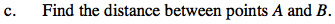Use the Pythagorean Theorem to find the distance.

Use the eTool below to help solve the problem.
Click the link at right for the full version of the eTool: GC 8-89 HW eTool